# An Alternative To The Sharpe Ratio

## Applications of Time Series Analysis (ARMA-GARCH) To Improve Risk-Adjusted Return Screening Procedures.

A naive approach might be to select the strategy with the best-annualized return, however, this ignores the level of potential risk that may materialize in future returns. For instance, suppose that the returns on Strategy A are better than that of Strategy B, but strategy A has a higher variance in daily returns (volatility). This means that a large loss in a single day is more likely for strategy A than strategy B.

A typical way that investors deal with this conundrum is to compute a measure of risk-adjusted return called the Sharpe Ratio. This is easy to calculate, simply take the average daily return “r” and divide by the standard deviation in daily returns “sigma”, also known as the volatility.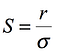The Sharpe Ratio (r = average daily return, sigma = standard deviation in daily returns). Some of you may immediately jump and say that I’ve forgotten to subtract the risk-free rate from the strategy returns, but these days it's so close to zero we can forget about it.

Thus we can now rescale the average daily returns of any strategy by its risk and directly compare any strategies by this standardized metric.

In theory this should be an excellent metric to solve our original problem, we can now declare the strategy with the best Sharpe Ratio as the best investment opportunity.

However, in practice, we recognize that the daily returns on any investment strategy are random quantities. This means that any sample statistics such as the average daily return or the volatility carry a level of uncertainty or noisiness. To see this simply compute the two values on different sections of the returns series say the first quarter and the second quarter of the year and the values of r and sigma won’t match.

Hence if both r and sigma are random variables the Sharpe ratio itself must also carry some uncertainty in its true value.

Clearly, this is problematic: Suppose two investment strategies have similar Sharpe ratios estimated from a small amount of historical data.

How confident can we be that one strategy is truly superior to the other or that either strategy will have a positive return in the long run?

This is now a problem for the statisticians, but fortunately, this is not too complicated so I will attempt to explain everything you need to know in simple terms and without too much algebra.

For many real-world quantities if we take a sufficiently large random sample from a population and plotted the frequency at which each value occurred in the sample we would get a bell curve shape which the stats people call a normal distribution. A simple example would be to randomly select and measure the heights of 100 individuals and plot the frequency of each height on the y axis and the corresponding height on the x-axis.

We can see we get this bell curve or “normal distribution” shape. Now replace the height variable with daily returns of a given investment strategy subtract the average daily and divide through by the standard deviation of those returns and we would get a centered “standard normal distribution” something like this.

Mathematically we can write the transformation of daily returns r_i to standardized returns z_i as follows:

As usual r and sigma take on the same meaning as in our Sharpe Ratio (avg daily return and volatility in daily returns). Additionally, we have written the symbols ~N(0,1) which says that the z_i’s are “distributed normally“ with mean zero and variance of one (this is called the standard normal distribution).

Assumption 1: The above statement is in fact in general not entirely correct as returns are not normally distributed, but if we take the log of daily returns they usually will be. We will however ignore this complication for now and assume what we have done is true.

Now let's do a simple little bit of manipulation of variables. Let's take for granted that if I multiply a normal random variable by x, then the mean distribution is scaled by x and its variance is scaled by x².

Applying this result we can rearrange the previous equation to obtain that our daily returns are normal random variables with mean r and volatility/variance sigma². (First, multiply z_i by sigma and then add r to get this result)

Assumption 2: Lets suppose that the daily returns are independent of each other and identically distributed with mean r and variance sigma². Again this is not true in general, if you’ve ever done any trading you’ll know that up days tend to follow up days and down days tend to follow down days. So clearly in the real world daily returns are correlated. But again lets assume this was true for a minute.

Using this assumption, we use another property that says when we add independent normal random variables together their variances and means add together.

Recall that the average daily return “r” is simply the sum of the daily returns divided by the number of returns we observed (let's call this number of days “n”). Then we can quickly see that the average return is also a normal random variable with mean r and variance sigma² over n (mean = n * r / n, variance = n * sigma²/n² = sigma²/n).

You can probably see where this is going, finally lets divide “r” by sigma and lone behold we have our Sharpe ratio again!

But wait a second now we see the Sharpe Ratio is in fact a random variable just as we claimed earlier on. Under our assumptions, it is in fact normally distributed with the mean equal to the Sharpe Ratio and Variance of 1/n.

Let's get some intuition here. Assuming all else equal if we sampled daily returns from different non-overlapping periods and computed the Sharpe Ratio in each period we could build a bell curve just like we did with returns. We would expect the bell curve to be centered around the “true” Sharpe ratio(S) with 95% of observed estimates lying between S - 2/n and S + 2/n.

Hence if we have an estimate of the Sharpe ratio from real data, we can be confident that 95% of the time the true Sharpe ratio will lie between our estimate S_hat -2/n and S_hat+2/n where n is the number of observed daily returns.

The more returns we have the larger “n” will be and thus the narrower our interval will become, or conversely, we will be highly uncertain about the claimed Sharpe of strategy with little historical return data.

In short if the confidence intervals of two strategies Sharpe ratios overlap then we cannot be confident that the future performance of strategy A will be significantly better than strategy B. Similarly if either strategy’s confidence interval contains zero, we cannot even be confident that in the long run we will make any money!

The key takeaway from this section is that we cannot trust the point estimate of the Sharpe ratio from a sample, we must be able to determine whether the noisiness of our estimate could include the possibility that the positive returns of a strategy are pure luck of the draw and not due to the skill of the fund manager.

## But what about all those assumptions you made earlier that you said were not true!

Actually, the vast majority of funds will quote only the point estimate of their Sharpe ratio, despite the fact that these estimates could be very noisy! Worse still they will use the assumption of independence (IID Assumption) to annualize the Sharpe ratio as follows:

1. Using the IID assumption we can add 252 daily returns together to get the annual return, we can ignore the compounding of returns because daily returns are usually tiny like 0.05% or something so it will be approximately the same. A quick proof for the interested using Taylor series expansions:

If you didn’t understand that it's easy to convince yourself this approximation holds in an excel spreadsheet.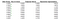You can see that the sum of returns approximates the true compound return, but the application of Taylor series approximation twice (as in the “Sum of Returns” column) is worse than only applying the approximation once (See “Exponential Approximation”)

2. Using the approximation that we can just sum over daily returns and use the fact that the sum over n elements IID random variables is equivalent to n times the mean value (on expectation), then we can obtain the following approximation for annualizing the Sharpe ratio.

Again I emphasize that this will give you misleading results, misuse of statistical assumptions like pretending that returns are independent will inflate the Sharpe ratio and also produce overconfidence (too narrow) intervals in which we believe the true value may lie.

## The Hidden Assumption

There is also another big assumption that snuck in the back door. By dividing through by sigma we implicitly are assuming that the volatility at every time point at which we observe returns is the same. This assumption is terrible, in fact, that there is an index called “The VIX” which tracks the changes in market volatility over time (The VIX will be higher during times of market volatility). If you need any further convincing about how bad this assumption is take a look at this chart which estimates the volatility of a randomly selected mutual fund’s daily returns. It's full of periodic spikes, rises, and falls (the large one is COVID-19).

## What impact might the failure of these assumptions have?

There are several potential consequences:

1. The Failing of IID Assumption will result in underestimation of the “constant” volatility and thus inflate the Sharpe ratio
2. Assumption of constant volatility means that returns that were achieved at lower volatility will be underweighted whilst returns that were achieved at a higher than estimated volatility will be overweighted. If there's a skew in returns (which also voids the normality assumption) then this will also likely skew the Sharpe ratio.
3. We can’t create a confidence interval making it impossible to compare two strategies reliably.

There are in fact some ways to adjust for the failing of the assumptions of the Sharpe Ratio described in “The Statistics of Sharpe Ratio’s” (Andrew W. Lo, 2002), which addresses the first issue of non-IID returns (IID = Independently Identically Distributed), but not the second of non-constant variance. The method described is quite involved especially when attempting to annualize the Sharpe Ratio correctly and not something mere mortals such as myself would feel comfortable implementing correctly (or understanding). So can we find a more statistically valid metric than the Sharpe Ratio without crazy complicated maths? The answer is yes and we can easily implement this in R or Python with a few lines of code.

## A Better Way (Enter Time Series Analysis…)

In general, the returns on financial time series data are correlated with previous values of the series and the volatility in returns are typically not constant as we have discussed. We can therefore model this behavior using an ARMA-GARCH model from time series analysis. One of the advantages of this model is that what is left after modeling out the above effects will be a white noise of a know distribution. Thus these residuals will have independence and hence provide a way to calculate a confidence interval that is statistically correct.

## What is an ARMA-GARCH Model

The ARMA (Auto Regressive Moving Average) part of the model says that if we observe return r_t at time t then it can be explained by a weighted sum of past values of the series and a weighted sum of the past realizations of random fluctuations (eta_t) in the time series which are assumed to be drawn from a known distribution (eg normal). Thus the ARMA part of the model takes care of autocorrelation in the series, fixing the IID issue we had with the Sharpe Ratio.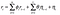ARMA(u,v) Process — Yes its as simple as it looks, the complicated bit is actually estimating the theta’s and phi’s but R will take care of that for you. Think of this just like a linear regression — but on the past randomness and values of the series rather than on other variables.

The GARCH (Generalised Auto Regressive Conditional Heteroskedasticity) part of the model says that the volatility of these random fluctuations is in fact a weighted sum of past shocks to the series and past volatilities. These volatilities are called “conditional volatilities” as they are a function of past realizations of the series and thus deals with the second failing of the Sharpe Ratio, which was the constant variance assumption.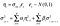GARCH(p,q) Model, the square of the volatility (variance) of the random terms are modeled as fluctuating and thus rescaling the white noise epsilon_t’s variance. Again think of the conditional variance as a regression on past shocks to the series and past conditional variances. Again RStudio (R; fGarch Library) will take care of estimating the alphas and betas.

Once we model these to components we can add in an estimate for the average daily return of the series which as usual we denote “r” which will be jointly estimated with all of the above effects modeled in, using the modification below.

As a result, the average daily return will be calculated simultaneously with all other model parameters and hence will not be biased by autocorrelation and non-constant volatility as these are baked into the modeling assumptions.

We can interpret this average daily return metric as the “de-risked” or “intrinsic” return we will obtain on average after modeling out all of the distortions due to volatility and autocorrelation risks. As an added benefit the intrinsic return will be from a known distribution and thus R will provide us with confidence intervals enabling comparison across different strategies.

# Estimating Intrinsic Returns of 500 Randomly Selected Mutual Funds

In an attempt to validate the theory I’ve come up with, I analyzed data for returns for 500 publicly traded mutual funds from December 2017 until December 2020. The funds were selected at random from a much larger database (So no Finite Population Correction required for standard errors). I then used an automatic fitting procedure based on the AIC information criteria to select the best model for each return series using only data from 2017–2018. I used a search space of ARMA(u, v) + GARCH(p, q) with u, v in [1,..,4] and p, q in [1,2] in order to fit the models in a reasonable amount of time on a laptop (overnight). Ideally, I would like to go through and check the assumptions of the model for each series individually but that would clearly be impractical at this sample size, so we will just run with the best model which should provide reasonable fits in most cases.

## Key Findings

In the above figure, I plot the estimated intrinsic return against the estimated Sharpe ratio. The key findings are the following:

## Intrinsic Return and Sharpe Ratio Positively Correlated

From the mini-plot we can see that the Intrinsic return and Sharpe ratio are positively correlated, which indicates that they measure roughly the same concept (“Risk-adjusted / De-risked return”). The relationship appears to be linear or possibly quadratic as there appears to be greater dispersion in intrinsic returns for positive Sharpe Ratios, so I will refrain from quoting linear correlation coefficients here.

## Sharpe Ratio Exhibits Overconfidence In Point Estimates Relative to Intrinsic Return Metric

On the main plot, I marked all mutual funds which had significant risk-adjusted returns for both the intrinsic return and the faulty Shape confidence intervals. Subsequently, I mark with a blue X those that were significant by Sharpe but not by Intrinsic. It is thus clear that there are many more funds that are significant under the faulty assumptions of the Sharpe ratio than under the confidence interval of the intrinsic return. In other words, the Sharpe Ratio assumptions lead to overconfidence in the point estimates closeness to the true risk-adjusted return.

## Sharpe vs Intrinsic as Predictor of Future Returns

In the below Figure, we plot the returns Post COVID (December 2020) grouped by whether the risk-adjusted return was significant by both (Sharpe + Intrinsic), either or neither metric. Note that this period is out of sample as we only estimate Sharpe ratio and Intrinsic Return up to December 2018.

I then perform a one-way ANOVA and find significant evidence that the mean return is different across the groups/treatments. Finally, I run a Games Howell test and find that there is only a significant difference in the means between the “Both” and “Insignificant” groupings, but not for other group comparisons. Hence this provides further evidence to suggest that the Sharpe Ratio is overly confident and that this issue can be rectified by also computing the Intrinsic return and using the two metrics significance as a joint criterion for identifying funds with significantly positive returns.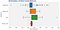Box plot of Post COVID (Out of Sample) Returns on 500 Mutual Funds, grouped by the significance of estimators in 2017–2018 Period.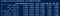One Way ANOVA + Games Howell Test

Code for Auto ARMA GARCH Fitting: Github Gist.

Code for Games Howell Test: Github Gist

You’ve been reading Quantamental the UCD Investors & Entrepreneurs Society Data Science and Financial Research Blog

Written by